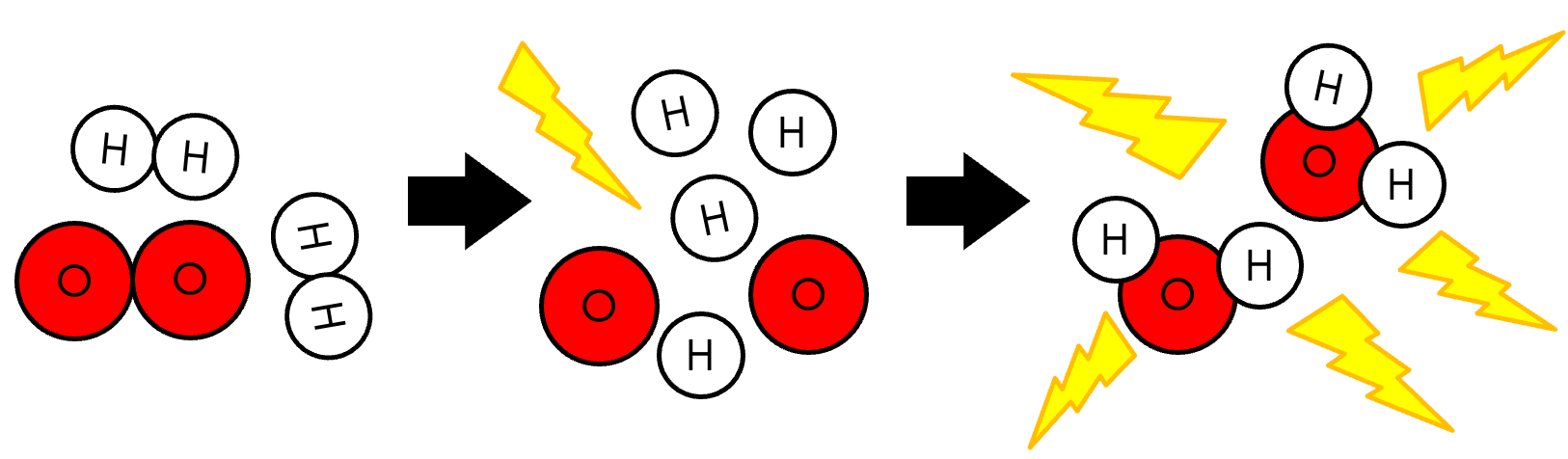# Synthesis and Decomposition

This is part of Year 11 HSC Chemistry course under the topic of Chemical Reactions

### HSC Chemistry Syllabus

• Conduct investigations to predict and identify the products of a range of reactions, for example:

– Synthesis

– Decomposition

### Synthesis and Decomposition Reaction

This video defines the distinguishing characteristics of two classes of reaction: Synthesis and Decomposition.

### Synthesis Reactions

A synthesis reaction is a reaction which involves the combination of chemicals to form a larger compound.

The general equation for synthesis reactions is given as:

$$A + B \rightarrow AB$$Beyond forming simple binary compounds like AB, synthesis can combine various elements and compounds to form larger structures. E.g.

$$AB + BC \rightarrow AB_2C$$

• Bond formation: Synthesis reactions are not limited by the bond types which are formed. Both ionic and covalent substances can be synthesised.

• Energy involved: Synthesis type reactions are exothermic in nature as they involve bond formations which are an exothermic process.

Example: Formation of Ammonia (Haber Process)

$$3H_2(g) + N_2(g) \rightarrow 2NH_3(g)$$
In this reaction, covalent bonds are formed between separated nitrogen and hydrogen particles.

Notice how this synthesis reaction involves both the formation and breaking of bonds. N-H bonds are formed, but the bonds between H_2 and N_2 must first be broken. If bond breaking is endothermic, and bond formation is exothermic, why then is this synthesis reaction still overall exothermic? This is because the bonds formed are stronger than those broken. As such, the bond formation step releases more energy than that initially used to break the reactant bonds.

Example: Formation of water from hydrogen and oxygen gas

$$2H_2(g) + O_2(g) \rightarrow 2H_2O(l)$$### Decomposition Reactions

Decomposition reactions are the opposite of synthesis reactions. They entail the breakdown of compounds into simpler products.

The general equation for decompositions reactions is given as the opposite of synthesis reactions:

$$AB \rightarrow A + B$$

• Bond breakage: Decomposition reactions like synthesis reactions, are not limited by the bond types which are formed. Both ionic and covalent bonds can be broken.

• Energy involved: Decomposition type reactions involve the breaking of bonds. Energy is required to be absorbed in order to break those bonds so decomposition reactions are endothermic.

Example: Decomposition of calcium carbonate

A common example of decomposition that will also be explored in year 12 HSC Chemistry is the thermal decomposition of calcium carbonate:

$$CaCO_3(s) \rightarrow CaO(s) + CO_2(g)$$

In this case, the decomposition of calcium carbonate involves the breaking and forming of both ionic and covalent bonds. The energy required to break bonds is greater than that released by the bonds formed. As such, the overall process is endothermic.

Example: Decomposition of AgCl

$$2AgCl(s) \xrightarrow{\text{UV light}} 2Ag(s) + Cl_2(g)$$

Photographic film utilises the decomposition of AgCl to capture and preserve images.• Bond breakage: Decomposition reactions like synthesis reactions, are not limited by the bond types which are formed. Both ionic and covalent bonds can be broken.

• Energy involved: Decomposition type reactions involve the breaking of bonds. Energy is required to be absorbed in order to break those bonds so decomposition reactions are endothermic.

Previous section: Physical and Chemical Change

Next section: Precipitation and Solubility Rules